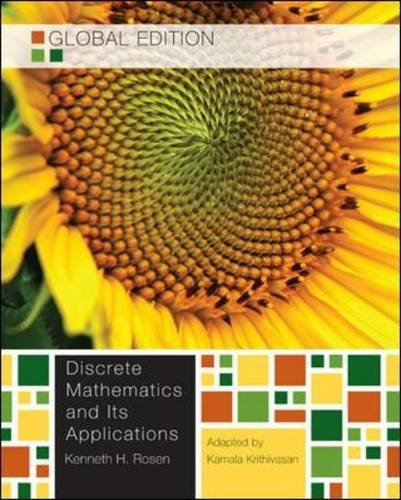HomeDepartmentUndergraduateGraduateResearchAccreditationsAlumni TürkçeCourse InfoAnnouncementsAssignments

# BIM 122 - Discrete Computational Structures

Instructor: Dr. Öğr. Üyesiİsmail SAN
Teaching Assistants:
Course Book:

Discrete Mathematics and Its Applications Global Edition, 7/e, by Kenneth Rosen and Kamala KrithivasanMidterm 2 : 20%
Quiz: 20%
Final : 40%
Other Resources:
Course Outline:
1. Week

Welcome to the Course!

• Introduction to BIM122

The Foundations: Logic and Proofs

• Propositional Logic
• Applications of Propositional Logic
 introduction_bim_122.pdf propositions.pdf

2. Week

The Foundations: Logic and Proofs

• Propositional Equivalences
• Predicate Logic and Quantifiers
 predicates_and_quantifiers.pdf prop_equiv.pdf

3. Week
• Predicate Logic and Quantifiers
• Nested Quantifiers

4. Week
• Rules of Inference
• Introduction to Proofs

5. Week
• Proofs
• Proof Strategies
 proofs_continued.pdf proofs_strategies.pdf

6. Week

Basic Structures:

• Sets
• Functions
• Sequences
• Sums
• Matrices

7. Week

Algorithms:

• Algorithms
• Growth of Functions
• Complexity of Algorithms

8. Week

Number Theory and Cryptography:

• Divisibility and Modular Arithmetic
• Integer Representations and Algorithms
• Primes and Greatest Common Divisors
• Solving Congruences
• Applications of Congruences
• Cryptography

9. Week

Boolean Algebra:

• Boolean Functions
• Representing Boolean Functions
• Logic Gates
• Minimization of Circuits
 Boolean_Algebra.pdf SupplementalChapter_BooleanAlgebra.pdf

10. Week

Modeling Computation:

• Finite State Machines with outputs
• Finite State Machines without outputs
• Turing Machine
 Modeling_Computation.pdf SupplementalChapter_ModelingComputation.pdf

11. Week

Graphs:

• Graphs and Graph Models
• Graph Terminology and Special Types of Graphs
• Representing Graphs